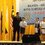# Help me with this.

Find minimum 2 digit n, such that $$(7^{n} + 7^{n-1} + 7^{n-2} + ...... + 7^{3} + 7^{2} + 7^{1} + 7^{0})$$ is a perfect square, since known $$(7^{3} + 7^{2} + 7^{1} + 7^{0})$$ is a perfect square.

Note : $n \neq 3$ !!!Note by Bryan Lee Shi Yang
6 years, 2 months ago

This discussion board is a place to discuss our Daily Challenges and the math and science related to those challenges. Explanations are more than just a solution — they should explain the steps and thinking strategies that you used to obtain the solution. Comments should further the discussion of math and science.

When posting on Brilliant:

• Use the emojis to react to an explanation, whether you're congratulating a job well done , or just really confused .
• Ask specific questions about the challenge or the steps in somebody's explanation. Well-posed questions can add a lot to the discussion, but posting "I don't understand!" doesn't help anyone.
• Try to contribute something new to the discussion, whether it is an extension, generalization or other idea related to the challenge.

MarkdownAppears as
*italics* or _italics_ italics
**bold** or __bold__ bold
- bulleted- list
• bulleted
• list
1. numbered2. list
1. numbered
2. list
Note: you must add a full line of space before and after lists for them to show up correctly
paragraph 1paragraph 2

paragraph 1

paragraph 2

[example link](https://brilliant.org)example link
> This is a quote
This is a quote
    # I indented these lines
# 4 spaces, and now they show
# up as a code block.

print "hello world"
# I indented these lines
# 4 spaces, and now they show
# up as a code block.

print "hello world"
MathAppears as
Remember to wrap math in $$ ... $$ or $ ... $ to ensure proper formatting.
2 \times 3 $2 \times 3$
2^{34} $2^{34}$
a_{i-1} $a_{i-1}$
\frac{2}{3} $\frac{2}{3}$
\sqrt{2} $\sqrt{2}$
\sum_{i=1}^3 $\sum_{i=1}^3$
\sin \theta $\sin \theta$
\boxed{123} $\boxed{123}$

Sort by:

CAN U WRITE A PROOF TO SUPPORT THIS

- 6 years, 2 months ago

There is no solution < 270.

- 6 years, 2 months ago

Are there any solutions bigger than 270?

- 6 years, 2 months ago

$n = 37$ satisfies!

- 6 years, 2 months ago

>> n = 4

>> while (((7(n+1)-1)/6)0.5 - int(((7(n+1)-1)/6)0.5)) != 0:

                     n = n+1


My code! *Easy code, though!

- 6 years, 2 months ago

No it doesn't. 21655801907853836686195357616408 is not a square number.

- 6 years, 2 months ago

@D G $\frac{{7}^{38}-1}{6} = 21655801907853831608163177358336 = {4653579472605344}^{2}$

- 6 years, 2 months ago

Sorry, it is +1, not -1.

- 6 years, 2 months ago

Oh maybe. My IDLE is just reversing it up. When I replace - with +, it shows the above value(which should come with -).

- 6 years, 2 months ago

http://www.wolframalpha.com/input/?i=%287%5E38+-+1%29%2F6+&dataset=

- 6 years, 2 months ago

There has to be a problem with my python idle, then. Thanks!

- 6 years, 2 months ago

Up till n=22, only n=0 and n=4 are perfect square according to what I get from TI -83+ calculator. After that I do not get accurate calculations.

- 6 years, 2 months ago

I am sorry, bcs. I have no answer.

- 6 years, 2 months ago

If you take $n=0$, then you expression will be equal to $1$ which is a perfect square. So $n=0$ can be the one value. Also try to use the latex coding in your notes and problems (now I've put latex in it) , if you need a guide for latex, you can find it just by clicking here .!!! @Bryan Lee Shi Yang

- 6 years, 2 months ago